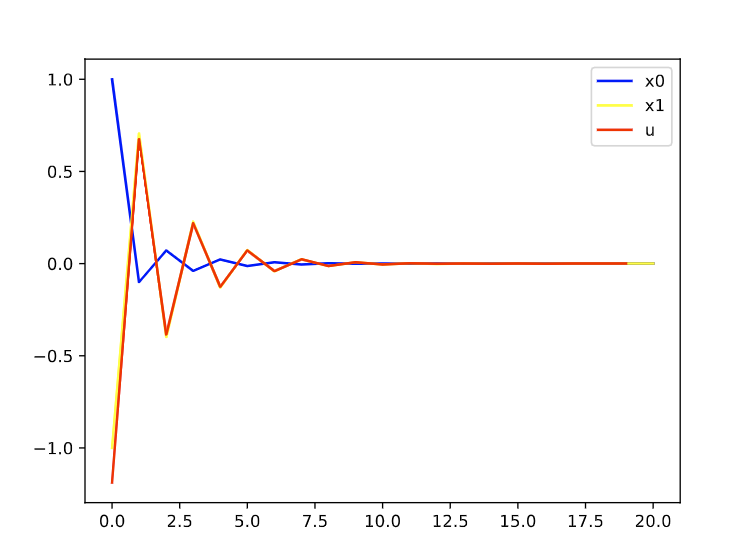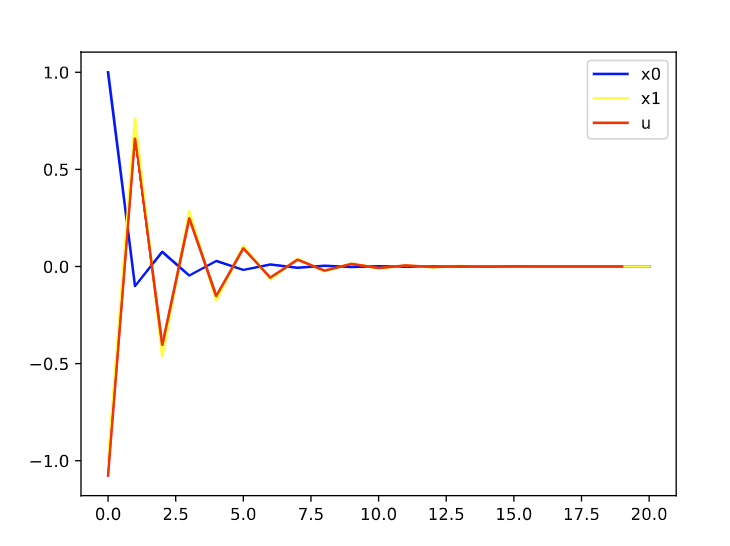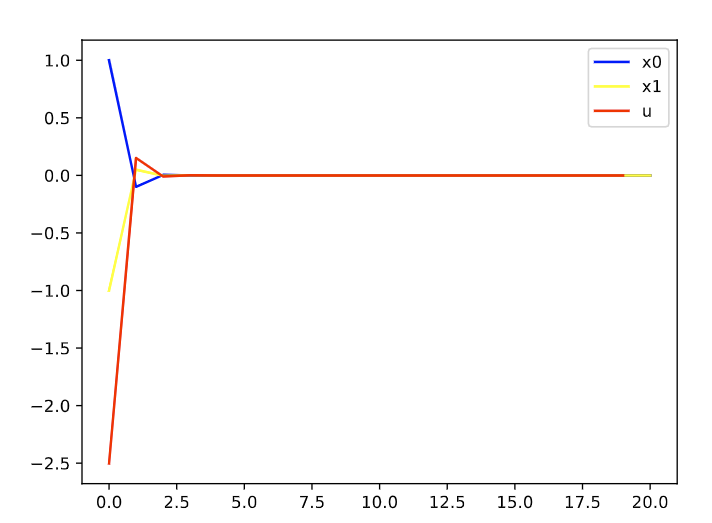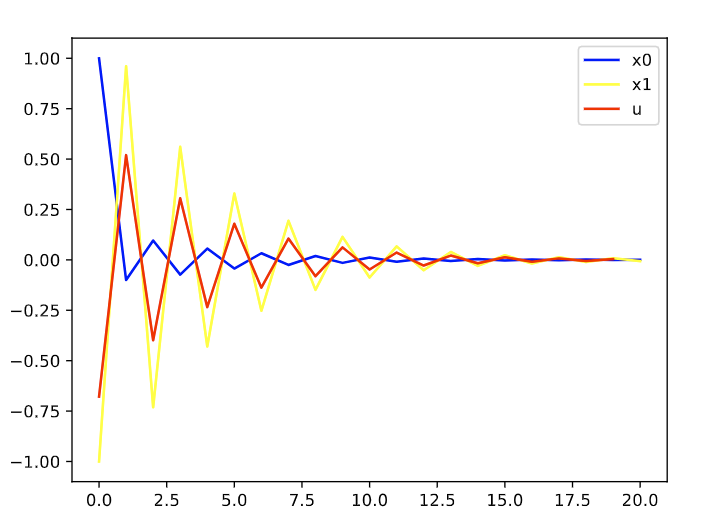0%

## MPC基本原理

MPC基本目标是保持在$$t$$时刻的状态接近于给定的trajectory，1950s开始，状态空间表示的系统开始流行，所以，当时LQR方法比较流行，取得了一些成果。可以，实际的系统往往是非线性的，且二次惩罚对于约束的处理有问题，不能保持硬约束。

$x_{k+1}=f_k(x_k, u_k)$

$$g_k(x_k, u_k) \geq 0, \quad for \quad all \quad (x_k, u_k)$$

$x_k \in X_k$, $$u_k \in U_k(x_k), \quad k=0,1,\cdots$$

$$f_k(0, \overline{u_k})=0,g_k(0,\overline{u_k})=0, \quad for \quad some \quad control \quad u_k \in U_k(0)$$

MPC的基本思想就是对于给定的初始状态$$x_0 \in X_0$$，我们想要获得一个控制序列$$u_0, u_1, \cdots$$，能够跟踪设定的轨迹，同时来使得状态和控制的约束满足，同时保证促使函数最小。

MPC具体算法流程：

Step1: 求解一个lookahead问题，需要$$x_{k+l}=0$$

Step2: 对于$$\{\overline{u_k}, \overline{u_{k-1}}, \cdots\}$$是计算出来的最优控制序列，只应用$$\overline{u_k}$$作为控制信号，舍弃剩下的控制信号

Step3: 在下一个时刻，MPC继续不断重复该求解过程

MPC基本理念

## 优化方法及框架

OSQP的文档中给出了一个MPC的demo，https://osqp.org/docs/examples/mpc.html

## 简单的代码实现

$$A=\left[\begin{array}{cc} 0 & 0.1 \\ 0.3 & -1 \end{array}\right]$$$$B=\left[\begin{array}{c} 0 \\ 0.5 \end{array}\right]$$

## 仿真验证控制时域和控制时域对控制器性能的影响

N=20, P=4时：N=30，P=6N=30，P=2N=30，P=1## MPC与RL的关系

If you like my blog, please donate for me.